Select Page

# CBSE MCQ Maths 12 Science Continuity and Differentiability Solutions in English

CBSE MCQ Maths 12 Science Continuity and Differentiability Solutions in English to enable students to get Solutions in a narrative video format for the specific question.

Expert Teacher provides CBSE MCQ Maths 12 Science Continuity and Differentiability Solutions through Video Solutions in English language. This video solution will be useful for students to understand how to write an answer in exam in order to score more marks. This teacher uses a narrative style for a question from Continuity and Differentiability not only to explain the proper method of answering question, but deriving right answer too.

Please find the question below and view the Solution in a narrative video format.

Question:

Solution Video in English:

You can select video Solutions from other languages also. Please check Solutions in ( Hindi )

## Similar Questions from CBSE, 12th Science, Maths, Continuity and Differentiability

Question 1 : Find the value of k, if the area of the triangle is 4 sq unit and vertices are (-2, 0), (0, 4), (0, k). (View Answer Video)

Question 2 : Findfor the function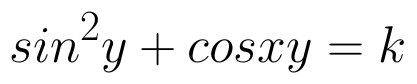. (View Answer Video)

Question 3 : Findfor the function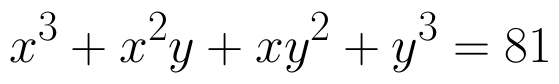. (View Answer Video)

Question 4 : Differentiate the function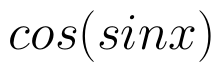with respect to x. (View Answer Video)

Question 5 : Findfor the function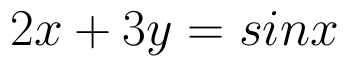. (View Answer Video)

### Determinant

Question 1 : If a, b, c are in AP, then determinant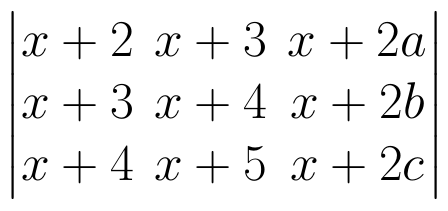. (View Answer Video)

Question 2 : The solution of the following system of equation is 2x + 3y = 5, 5x – 2y = 3. (View Answer Video)

Question 3 : Find the inverse of the matrix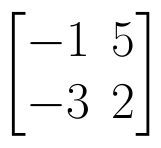. (View Answer Video)

Question 4 : A system of linear equations AX = B is said to be inconsistent, if the system of equations has, (View Answer Video)

Question 5 : If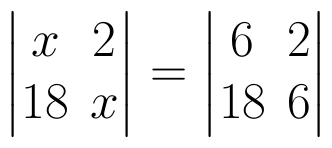, then x is equal to, (View Answer Video)

### Differential Equations

Question 1 : Form the differential equation of the family of circles touching the x-axis at origin .  (View Answer Video)

Question 2 : Find the particular solution of the differential equation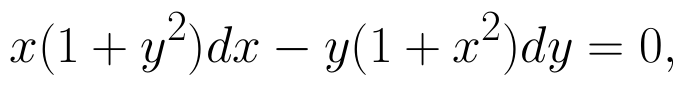given that y = 1, when x = 0. (View Answer Video)

Question 3 : Solve the differential equation: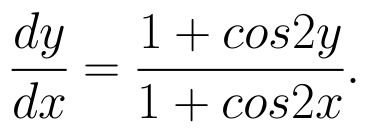(View Answer Video)

Question 4 : If x cos(a + y) = cos y, then prove that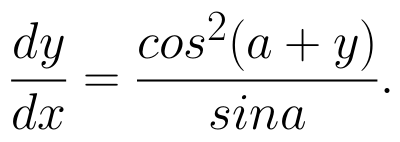Hence show that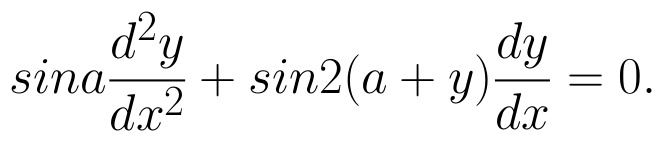(View Answer Video)

Question 5 : Find the general solution of differential equation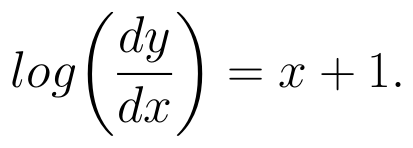(View Answer Video)

### Linear Programming

Question 1 : The objective function is maximum or minimum, which lies on the boundary of the feasible region. (View Answer Video)